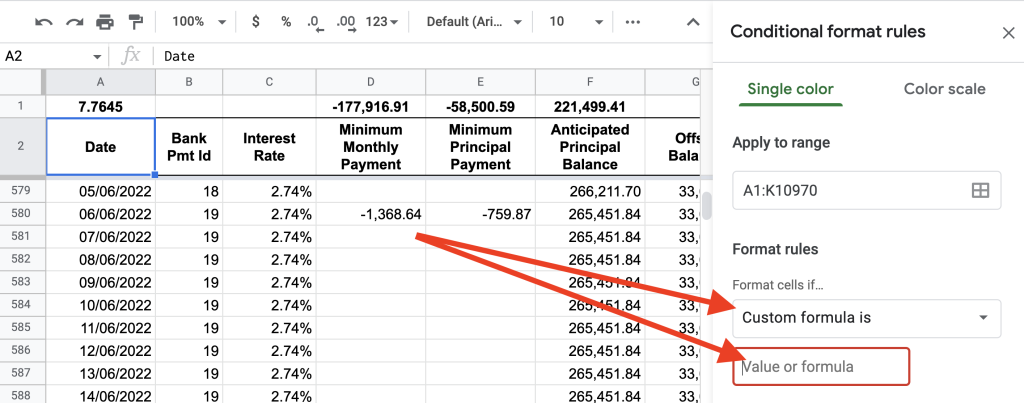# Apply Conditional Formatting To Entire Row: Google Sheets

How can you highlight an entire row based on a single condition in another column?

To highlight an entire row based on a value in a column using conditional formatting requires using the ``` INDIRECT() ``` formula.

A spreadsheet contains the following simple data where the first column contains a list of dates and the other columns contain corresponding data for that date.

Here’s a snapshot of the spreadsheet which contains ``` Date ``` , ``` Bank Pmt Id ``` and ``` Interest Rate ``` :

The simple spreadsheet has a payment schedule for each calendar day of the year and the corresponding interest charge, rates, principal amount and balances.

To highlight an entire row to check how the repayments are going a conditional format was placed on the entire sheet.

To do this, first highlight all cells in the active sheet.

Then click on the menu item Format then Conditional formatting .

With the conditional formatting sidebar that pops up click on the first dropdown menu labelled ``` Format cells if... ``` and select ``` Custom formula ``` :Click on the dropdown box which should enable you to enter a custom formula in an input box

As previously taught regarding conditional formatting and custom formulas the principles from this article help in easily creating a formula matching the requirement.

The custom formula is:

``=INDIRECT("A"&ROW(INDIRECT("RC", FALSE))=TODAY()``

The custom formula for the conditional format contains two ``` INDIRECT() ``` formulas. The inner ``` INDIRECT("RC", FALSE) ``` formula represents the active cell as it loops through the entire highlighted range.

The outer ``` INDIRECT("A"&ROW(x)) ``` formula references the required first column for each row so that the comparison can be made.

Finally, the condition ``` x=TODAY() ``` checks if the value in column A matches today’s date. If this is ``` TRUE ``` then the conditional formatting will apply the formatting set.

As you can see the custom formula inserted into the custom formula input box produces the highlighted row as it matches today’s date (don’t use ``` NOW() ``` as this includes time).

## Summary

To create a conditional format that highlights an entire row based on a column in that range meeting a specific condition use the ``` INDIRECT() ``` formula to first grab the active cell (using ``` INDIRECT("RC", FALSE) ``` ) and then use that in an outer ``` INDIRECT() ``` function to reference the specific column name along with ``` ROW(x) ``` .

As the condition for this specific requirement was to have the first column in the sheet match today’s date the custom formula ended up being:

``=INDIRECT("A"&ROW(INDIRECT("RC", FALSE)))=TODAY()``

Author of scripteverything.com, Ryan has been dabbling in code since the late '90s when he cut his teeth by exploring VBA in Excel when trying to do something more. Having his eyes opened with the potential of automating repetitive tasks, he expanded to Python and then moved over to scripting languages such as HTML, CSS, Javascript and PHP. When he is not behind a screen, Ryan enjoys a good bush walk with the family during the cooler months, and going with them to the beach during the warmer months.